Support the Monkey! Tell All your Friends and TeachersHome MonkeyNotes Printable Notes Digital Library Study Guides Study Smart Parents Tips College Planning Test Prep Fun Zone Help / FAQ How to Cite Request a New Title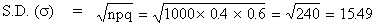Expected number = n p = 0.4 ´ 1000 = 400

Example Six dice are thrown 729 times. How many times do you expect at least three dice to show 5 or 6?

Solution : Let P = probability (showing 5 or 6) = 2/6 = 1/3

q = 1 - p = 1- 1/3 = 2/3

n = 6 and r = 3

Also p (x = r) = probability (at least 3 dice will show 5 or 6 in one trial)

Using the 'complement' theorem

p (x = r) = 1 - [p (x = 0) + p (x = 1) + p (x = 2)]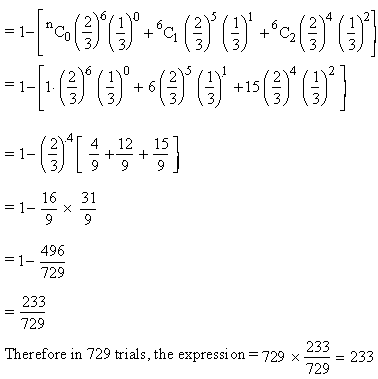Therefore in 729 trials, the expression =Your browser does not support the IFRAME tag.

Example Take 100 sets of tosses of 10 flips of a fair coin. In how many cases do you expect to get 7 heads at least ?

Solution : We have N = 100 sets. n = 10 trials in each set p = 0.5 and q = 1 - p =0.5
Probability (getting at least 7 heads) in one set

= p (x = 7) + p (x = 8) + p (x = 9) + p (x = 10)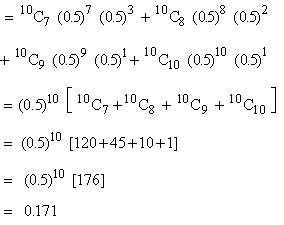Therefore in 100 sets = N p (r) = 100 ´ (0.171) @ 17 times you can expect to get at least 7 heads.

Example If the probability of success is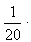How many trials are required in order that the probability of getting at least one success, is just greater than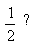Solution :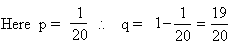Let 'n' be the required number of trials to get the probability of at least one success which is ,

1 - n C 0 P 0 Q n-0 \ [ since probability (at least one success) = 1 - p (x = 0)

i.e. 1 - probability (No success)]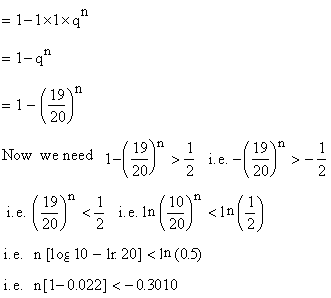Index

7. 1 Introduction
7. 2 Trial
7. 3 Sample Space
7. 4 Definition of Probability
7. 5 The Laws of Probability
7. 6 Conditional Probability
7. 7 Theoretical Distribution
7. 8 Binomial Distribution
7. 9 Normal Distribution
Chapter 8Search: All Products Books Popular Music Classical Music Video DVD Toys & Games Electronics Software Tools & Hardware Outdoor Living Kitchen & Housewares Camera & Photo Cell Phones Keywords: